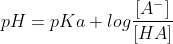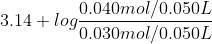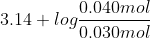In: Chemistry

# What is the pH of a buffer that is prepared by mixing 30.0 mL of 1.0...

What is the pH of a buffer that is prepared by mixing 30.0 mL of 1.0 M HF and 20.0 mL of 2.0 M KF? (K a a for HF 7.2 x 10 −4 )

## Solutions

##### Expert Solution

HF is a weak acid and KF is a salt which dissociates to give F-

which is the conjugate base of HF.

Also 1 L=1000 mL

As 1 mol KF gives 1 mol F- in solution

So Number of moles of F​​​​​​-=Numbe of moles of KF=Molarity x Volume =2.0 M x 20.0 mL/1000 mL/L=0.040 mol

Number of moles of HF=Molarity x Volume (L)=1.0 M x 30.0 mL/1000 mL/L

=0.030 mol

Total volume of the buffer=20.0 mL+30.0 mL=50.0 mL/1000 mL/L=0.050 L

By Henderson-Hasselbalch equation, pH of an acidic buffer is given asWhere Ka=acid dissociation constant for HF=7.2x10-4

pKa=-logKa=-log(7.2x10-4)=3.14

[A​​​​​-]=Concentration of conjugate base=concentration of F-=Number of moles of F​​​​​-/volume of the solution=0.040 mol/0.050 L

[HA]=concentration of weak acid=concentration of HF=Number of moles of HF/volume of the solution

=0.030 mol/0.050 L

Substituting the values in Henderson-hasselbalch equation

pH===3.14+0.12=3.26

So pH of given buffer=3.26

## Related Solutions

##### What is the pH of a buffer that is prepared by mixing 30.0 mL of 1.0...
What is the pH of a buffer that is prepared by mixing 30.0 mL of 1.0 M HF and 20.0 mL of 2.0 M KF? (Ka for HF 7.2 x 10−4) What is the pH of a solution that contains 0.40 M CH3COOH and 0.30 M CH3COONa at 25°C (Ka=1.8 x 10−5)?
##### What is the pH of a buffer prepared by mixing 20.00 mL of 0.0300 M ammonium...
What is the pH of a buffer prepared by mixing 20.00 mL of 0.0300 M ammonium chloride with 40.00 mL of 0.0450 M ammonia? What is the resulting pH if 1.00 mL of 0.10 M HCl is added to this solution?
##### What is the pH of a solution prepared by mixing 25 ml of 0.5 HCN with...
What is the pH of a solution prepared by mixing 25 ml of 0.5 HCN with 75 ml of 0.20 NaCN. Ka = 6.2*10^-10 for HCN. Hint: remember to dilutions first.
##### Calculate the pH of a buffer solution prepared by mixing 1.35mL of concentrated ammonia (14.8M) with...
Calculate the pH of a buffer solution prepared by mixing 1.35mL of concentrated ammonia (14.8M) with 1.07 g of solid ammonium chloride in a 1.00-L volumetric flask. The acid ionization constant of the ammonium ion is 5.6×10-10.
##### A buffer solution was prepared by mixing 300.0 mL of a 0.200 M solution of hydroxylamine...
A buffer solution was prepared by mixing 300.0 mL of a 0.200 M solution of hydroxylamine (OHNH2, Kb = 1.1 × 10-8) and 250.0 mL of a 0.300 M solution of its hydrochloride salt (OHNH3+ Cl- ). Calculate the pH of the solution after the addition of 1.08 g of solid NaOH (molar mass = 40.00 g/mol). Assume that there is no volume change upon the addition of solid. A. 8.22    B. 5.55 C. 6.30 D. 5.78
##### A buffer solution was prepared by mixing 300.0 mL of a 0.200 M solution of hydroxylamine...
A buffer solution was prepared by mixing 300.0 mL of a 0.200 M solution of hydroxylamine (OHNH2, Kb = 1.1 × 10-8) and 250.0 mL of a 0.300 M solution of its hydrochloride salt (OHNH3+ Cl- ). Calculate the pH of the solution after the addition of 1.08 g of solid NaOH (molar mass = 40.00 g/mol). Assume that there is no volume change upon the addition of solid.
##### (4). Chapter 14 Calculate the pH of the buffer prepared by mixing (a)  2.0 g of NaOH...
(4). Chapter 14 Calculate the pH of the buffer prepared by mixing (a)  2.0 g of NaOH with 12 g of NH4Cl and diluting to 500 ml with wate (b) 5.0 ml of 6.0 M HCl with 5.0 g of sodium acetate and diluting to 500 ml with water
##### A buffer solution was prepared by mixing 516.9 mL of 0.25 M acetic acid and 103.8...
A buffer solution was prepared by mixing 516.9 mL of 0.25 M acetic acid and 103.8 mL of 0.89 M sodium acetate. Calculate the pH of the solution given that Ka is 1.8 x 10—5 . Answer in 2 decimal places.
##### 1) A buffer is prepared by mixing 152 mL of 0.452 M HCl and 0.495 L...
1) A buffer is prepared by mixing 152 mL of 0.452 M HCl and 0.495 L of 0.400 M sodium acetate. a. What is the pH? b. How many grams of KOH must be added to 0.500 L of the buffer to change the pH by 0.18 units? 2) A buffer is prepared by mixing 52.6 mL of 0.050 M sodium bicarbonate and 10.0 mL of 0.10 M NaOH. a. What is the pH? b. How many grams of HCl...
##### 11. Calculate the pH of a solution prepared by mixing a) 48.0 mL of 0.212 M...
11. Calculate the pH of a solution prepared by mixing a) 48.0 mL of 0.212 M NaOH and 66.0 mL of 0.187 M HCl b) 69.0 mL of 0.345 M KOH and 125 mL of 0.400 M HC3H5O2 c) 350 mL of 0.130 M NH3 and 400 mL of 0.100 M HCl